Next: Example 2: Binomial distribution Up: Theoretical discrete distributions Previous: Theoretical discrete distributions   Contents

### Example 1: Bernoulli distribution

A Bernoulli (binary) variable is a random variable that can take only the value of either 1 (success) or 0 (failure). Bernoulli variables are commonly used for describing binary processes such as coin tossing, rain/no rain, yes/no decisions etc.. The Bernoulli distribution uses one population parameter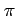to define the probability of success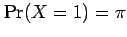and the probability of failure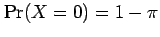. This can be written more succinctly as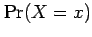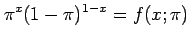(4.1)

where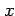takes the value of either 0 or 1. The parametercompletely determines the population distribution and all possible statistics based on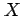, for example, the population mean is given by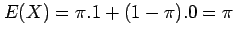and the population variance is given by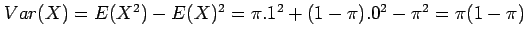. A random variabledistributed with a Bernoulli distribution is described as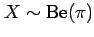by statisticians (the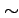symbol means distributed as'').

David Stephenson 2005-09-30